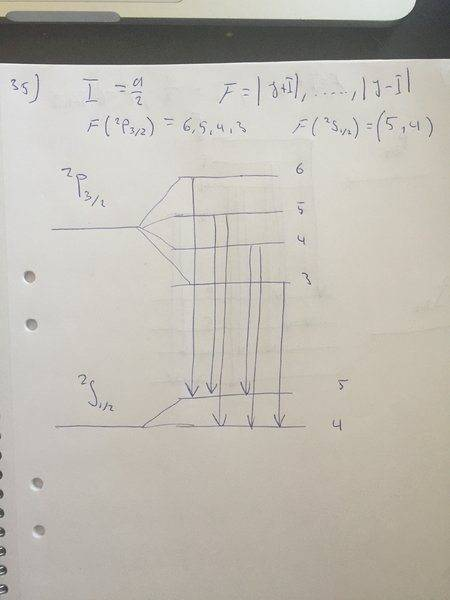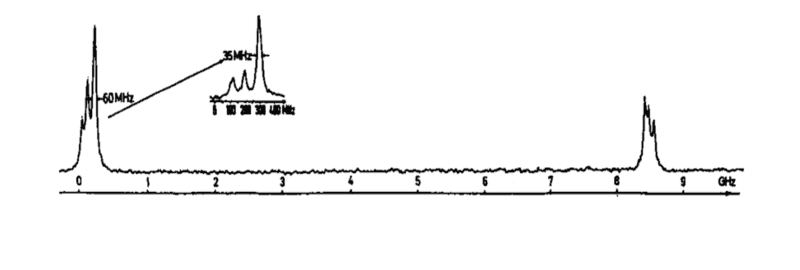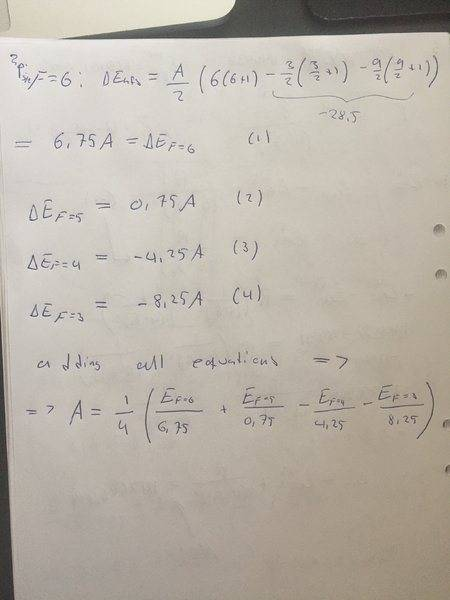# Determine fine-structure constant

## Homework Statement

The figure below shows the hyperfine structure in the transition 6s $^2S_{1/2}$ - 8p $^2P_{3/2}$ in 115In (I = 9/2). The measurement is made using a narrow-band tunable laser and a collimated atomic beam; hence the Doppler width is greatly reduced. The 6 components shown have the following frequencies 31, 112, 210, 8450, 8515 and 8596 MHz. Draw a schematic figure of the energy levels with the appropriate quantum numbers and show the allowed transitions. Determine the hyperfine constants, in MHz, for the two fine structure levels

(Image is just a blurry graph and not necessary, all data is given)

## Homework Equations

$$E_{hfs}=\frac{A}{2}[F(F+1) - J(J+1)-I(I+1)] (1)$$

## The Attempt at a Solution

F=|J+I|,....,|J-I|. For the dubblet P that would be F=(6,5,4,3). So I have F,J and I.

Since I don't have energydifferences (I think), I'm not sure how to put up a equationsystem to solve for (1). Are there another formula/concept I should use instead?

*Note I'm a beginner at atomic physics, brand new to these concepts so maybe I'm missing something obvious*

kuruman
Homework Helper
Gold Member
2021 Award
Hi Philip Land and welcome to PF.Drawing the energy level diagram and identifying the transitions would be extremely useful as a first step. Have you done that? If "yes", please post the drawing; if "no", please do it.

On edit: Please post the graph that you think is "not necessary". Also, if you think that "all data is given", why then do you think that you "don't have the energy differences"?

Last edited:
Hi Philip Land and welcome to PF.Drawing the energy level diagram and identifying the transitions would be extremely useful as a first step. Have you done that? If "yes", please post the drawing; if "no", please do it.

On edit: Please post the graph that you think is "not necessary". Also, if you think that "all data is given", why then do you think that you "don't have the energy differences"?

Hi!

I did as you said and drew the energy diagram. See uploaded picture. Also a print-screen of the graph belonging to the exercise.

You are right, I do probably have the energy differences in MHz, but I'm not quite sure how to identify the transitions.

I also tried to use formula (1), with the biggest frequency, 8596MHz on the left hand side, and used $F_{max}$ as my F in the equation. But that was a bold guess and the the answer got wrong.

So now I'm pretty stuck again, I know there is the Landé interval rule stating $$Energy ratio = \frac{F_{max}}{F_{max}-1} (2)$$ but I can't see how that can help me determine A either...#### Attachments

Last edited:
mjc123
Homework Helper
OK, so you have the a diagram of the energy levels and the transitions. Now use your equation for Ehfs to obtain values for the energy levels in terms of A (remember the A values will be different for the 6s and 8p states, you are asked to find both). Assume a value T (unknown) for the energy difference between the unsplit S and P states. Now derive expressions for the energies of the allowed transitions. Do you observe any patterns that would help you assign the observed frequencies to particular transitions?

OK, so you have the a diagram of the energy levels and the transitions. Now use your equation for Ehfs to obtain values for the energy levels in terms of A (remember the A values will be different for the 6s and 8p states, you are asked to find both). Assume a value T (unknown) for the energy difference between the unsplit S and P states. Now derive expressions for the energies of the allowed transitions. Do you observe any patterns that would help you assign the observed frequencies to particular transitions?

Hi!

I see. Starting with ##P_{3/2}##, I sat up 4 equations for all the F values (6,5,4,3). Then I solved for A (see attached picture).

Now I guess all I need to do is to figure out what ##E_{F=i}## for i=(6,5,4,3). I tried to use them in magnitude order, but the result got plain wrong.

Am I on the right track or am I thinking about it wrong? Because I really can't see the connection between the given frequencies and ##E_{hfs}##.#### Attachments

mjc123
Homework Helper
The frequencies aren't directly connected to Ehfs. They are transition frequencies, so refer to the difference between two energy levels. Repeat what you've done for the 2S level, and work out the energies of the allowed transitions.

The frequencies aren't directly connected to Ehfs. They are transition frequencies, so refer to the difference between two energy levels. Repeat what you've done for the 2S level, and work out the energies of the allowed transitions.
Are you suggesting that I multiply with planks constant and change unit? Or do you mean I should do something else?

I see, thanks. Can you give me a hint on how I work out these energies so I can solve for A? And do you mean I need to repeat the same doe ##^2S## before working out the energies to determine A for ##^2P##?

mjc123
Homework Helper
Are you suggesting that I multiply with planks constant and change unit?
No, use MHz as your "energy" unit, as you are given the frequencies in MHz and asked for the A values in MHz.
And do you mean I need to repeat the same doe 2S2S^2S before working out the energies to determine A for 2P2P^2P?
Yes. You need to obtain expressions for the TRANSITION energies. You can't work out A (2P) just from the expressions for the 2P energy levels, because you have no (direct) data on the relative energies of these levels. You have frequencies for the transitions between the 6s and 8p levels.

No, use MHz as your "energy" unit, as you are given the frequencies in MHz and asked for the A values in MHz.

Yes. You need to obtain expressions for the TRANSITION energies. You can't work out A (2P) just from the expressions for the 2P energy levels, because you have no (direct) data on the relative energies of these levels. You have frequencies for the transitions between the 6s and 8p levels.

I see. Sorry for being confused, this is a central part but not covered in lectures or textbook and I really want to grasp this.

I now have the expressions $$A_{^2P} = \frac{1}{4}[ \frac{E_6}{6.75} + \frac{E_5}{0.75} - \frac{E_4}{4.25} - \frac{E_3}{8.25} ] (1)$$
$$A_{^2S} = \frac{1}{2}[ \frac{E_5}{2.25} - \frac{E_4}{2.75} ] (2)$$

Is it so that the frequencies now is the energy-differences in these expressions, e.g. ##E_6 - E_5## is the given frequencies. I'm still not sure how I know what frequency correspond to what energy differences. Nor how to solve the equation system above. Do you have a lsat hint on this so it clicks?

mjc123
•